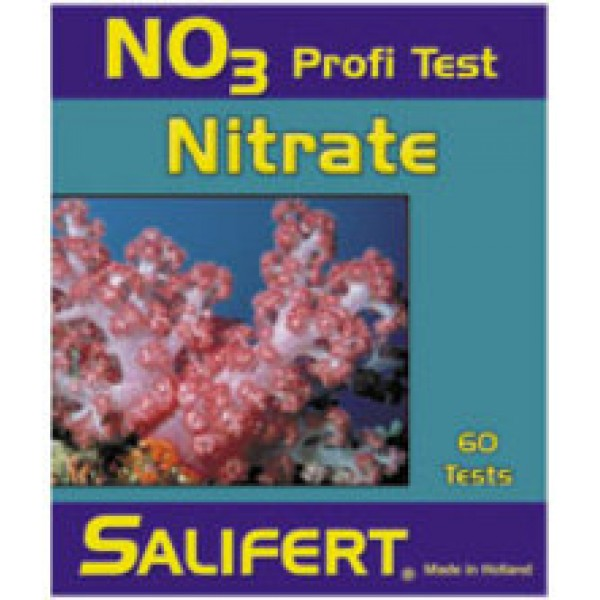# Salifert Nitrate Test Kit

Regular price \$18.95 Sale

<p" align="left">When an aquarium has insufficient regions deprived of oxygen (= anaerobic zones) or denitrification proceeds incompletely then nitrate will build-up.

<p" align="left">A too high nitrate concentration can result in unwanted algae-growth and these might irritate corals in their neighborhood. <p" align="left">Nitrate as such also retards coral growth. <p" align="left">The nitrate concentration in a proper functioning reef aquarium should preferably be lower than 1 mg/L. Fish-only aquariums would usually show much higher nitrate concentrations. <p" align="left">Most test kits use a procedure in which a certain part of nitrate is converted chemically into nitrite and is measured as such. <p" align="left">Once again, just as with nitrite testing, amine interference has a major impact on the measured value. If such an interference occurs concentrations much lower than actual will be measured. <p" align="left">  <p" align="left">Conclusion: <p" align="left">Many nitrate test kits are prone to amine interference. This can give results much lower than actual. <p" align="left">The Salifert nitrate testdoes not suffer from such an amine interference. The very special ingredients allow a very fast and precise measurement.

The range spans from very low to a very high nitrate concentration (approx. 0.05 – 20 mg/L as Nitrate-Nitrogen or 0.2 – 100 mg/L as nitrate ion).

<p" align="left"><p" align="left"><p" align="left"><p" align="left"><p" align="left"><p" align="left"><p" align="left"><p" align="left"><p" align="left"><p" align="left">The kit can perform approx. 60 measurements. <p" align="left">